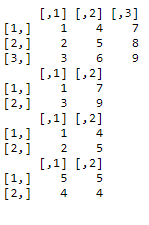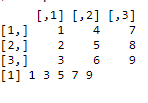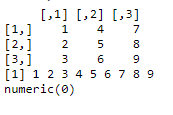Related Articles

# Get element at the specific position from matrix in R

• Last Updated : 07 Apr, 2021

At any point in time, a matrix may be required to be traversed for an element at a specific position. In this article, we are going to access the elements from a matrix in R Programming Language using integer vector, logical vector as the index.

### Method 1: Accessing elements using integer vector

Integer vector is a vector, that includes all elements of integer type.

Syntax:

matrix_name[row_vector_with_values,column_vector_with_values,]

Example:

select rows 1 & 3 and columns 1 & 3 of matrix a.

print(a )

Program:

## R

 `# create a vector named data with 9 elements``data=``c``(1,2,3,4,5,6,7,8,9)`` ` `# pass this vector to matrix input``a=``matrix``(data, nrow = 3, ncol = 3)``print``(a)`` ` `# select rows 1 & 3 and columns 1 & 3``print``(a[``c``(1,3),``c``(1,3)] )   `` ` `# select rows 1 & 2 and columns 1 & 2``print``(a[``c``(1,2),``c``(1,2)] )   `` ` `# select rows 2 & 1 and columns 2 & 2``print``(a[``c``(2,1),``c``(2,2)] )   `

Output:### Method 2: Accessing matrix elements using logic vector

Logical vector includes a vector comprising boolean values i.e. TRUE or FALSE.

Syntax :

matrix_name[logical_vector]

If TRUE at that position, matrix element is accessed

If FALSE at that position, matrix element is not accessed.

Example:

data=c(TRUE,TRUE,FALSE)

Program 1:

## R

 `# create a vector named data with 9 elements``data=``c``(1,2,3,4,5,6,7,8,9)`` ` `# pass this vector to matrix input``a=``matrix``(data, nrow = 3, ncol = 3)``print``(a)`` ` `a[``c``(``TRUE``, ``FALSE``,``TRUE``, ``FALSE``,``TRUE``, ``FALSE``,``TRUE``, ``FALSE``,``TRUE``)]``# accessing elements `

Output:Program 2:

## R

 `# create a vector named data with 9 elements``data=``c``(1,2,3,4,5,6,7,8,9)`` ` `# pass this vector to matrix input``a=``matrix``(data, nrow = 3, ncol = 3)``print``(a)`` ` `print``(a[``c``(``TRUE``)])``# accessing elements by placing all TRUE`` ` `print``(a[``c``(``FALSE``)])``# accessing elements by placing all FALSE`

Output:My Personal Notes arrow_drop_up